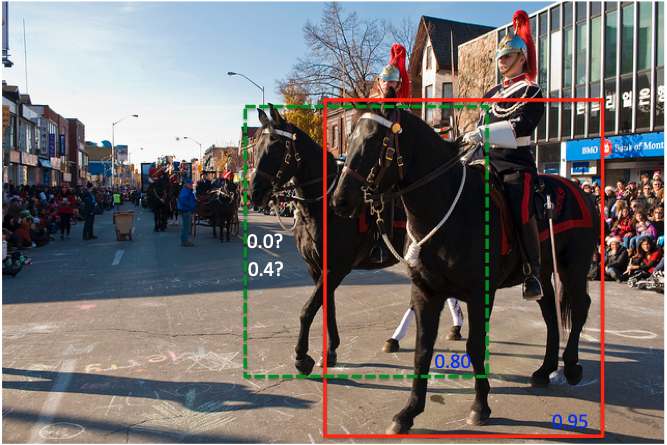# Soft NMS¶

## Soft NMS 提出背景¶

NMS（非极大值抑制）方法是目标检测任务中常用的后处理方法，其基本思想是：如果有多个预测框都对应同一个物体，则只选出得分最高的那个预测框，剩下的预测框被丢弃掉。在这种方法的处理下，可以有效地减少冗余的检测框。但是，传统的 NMS 算法会存在以下缺点：IOU阈值难以确定，阈值太小，则容易发生漏检现象，当两个相同类别的物体重叠非常多的时候，类别得分较低的物体则会被舍弃；阈值太大，则难以消除大部分冗余框。

## Soft NMS 算法流程¶

$\begin{split} s_i = \{\begin{matrix} s_i,iou(M,b_i)<N_t\\ 0,iou(M,b_i)\ge N_t \end{matrix} \end{split}$

$\begin{split} s_i = \{\begin{matrix} s_i,iou(M,b_i)<N_t\\ s_i(1-iou(M,b_i)),iou(M,b_i)\ge N_t \end{matrix} \end{split}$

## Soft NMS 算法示例¶